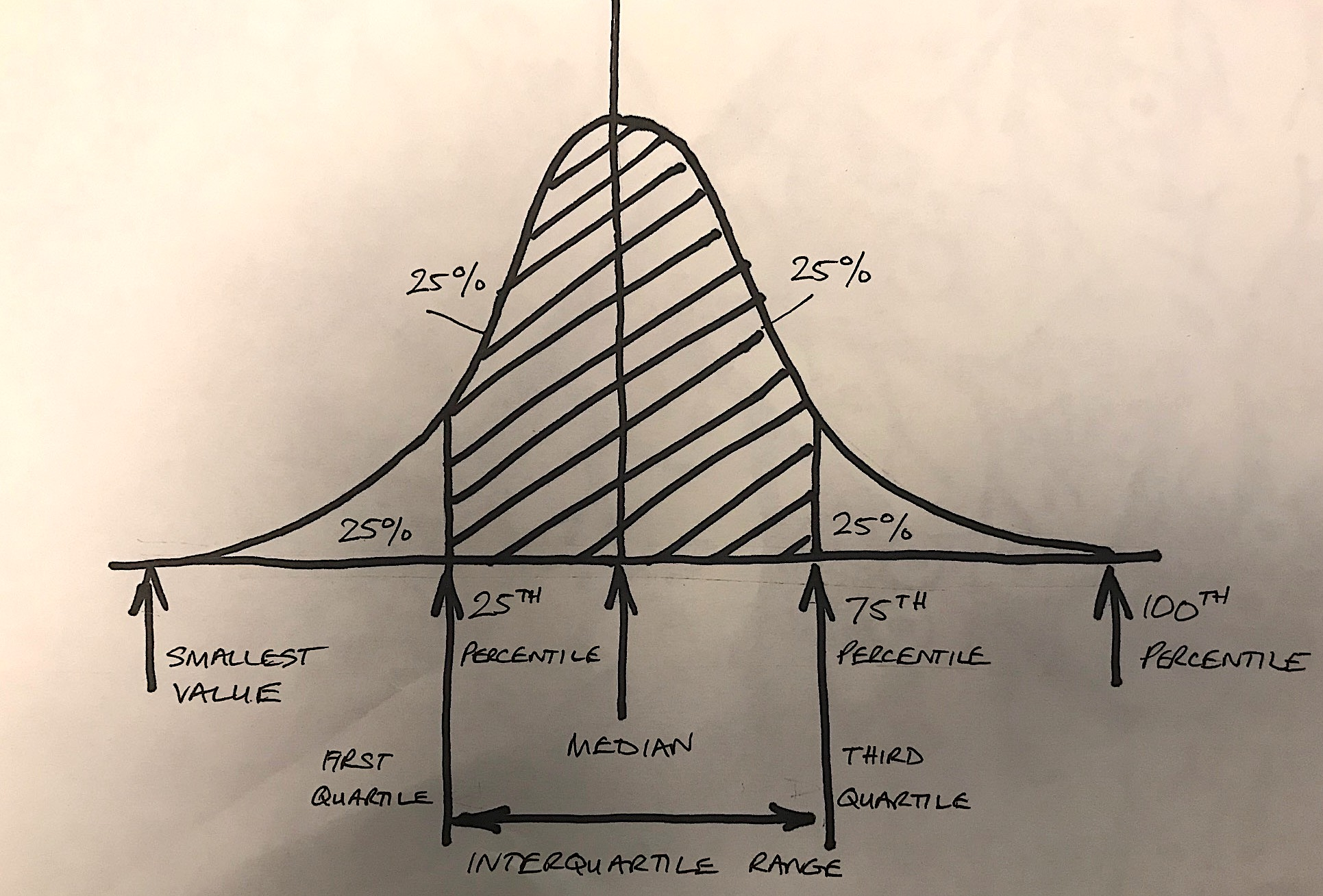# Statistics quantiles

Quantiles are useful for talking about where a particular value lies within a dataset. Unfortunately, they are not that widely understood.

We can think of quantile as equal portions of the data distribution. Once we have ordered the data, we can then split it up into different parts (tiles).

The first quarter of the data is referred to as the first quartile.

If we add another 25% of the data to the first quartile, we get the second quartile.

The third quartile contains the first 75% of the ordered data.

We can subtract the second quartile from the third quartile to find the interquartile range. This is the central 50% of the distribution.Sometimes you will hear percentile  or decile used when describing data. The percentile represents the proportion of the data that lies beneath an observed value. So, the 50th percentile is the values below which 50% of the data lies. Similarly, the 10th percentile represents 10% of the data.

The first quartile lies at the 25th percentile, the second quartile at 50% and the third quartile at 75%.

#### Be the first to commenton "Statistics quantiles"

This site uses Akismet to reduce spam. Learn how your comment data is processed.